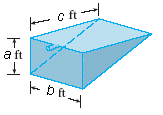# Work: Tank Of Water

RedBarchetta

## Homework Statement

A tank is full of water. Find the work W required to pump the water out of the spout. Use the fact that water weighs 62.5 lb/ft3.
a = 5
b = 9
c = 12## The Attempt at a Solution

So for this one, you have to set up a formula for a rectangular box of water.

The biggest rectangle is 5(or y) by 9, in relation to a smaller rectangle that is w by 9. I can also setup a relationship between the two to find that y/w=9/9 or y=w. So therefore we can produce an equation for the area of one rectangle.

dA=9y

Now the thickness of this rectangle is extremely small so the thickness is dy.

dV=9y dy

The density of water is 62.5 lb/ft^3, so this one rectangular-box of water is:

dM=562.5y dy

To find the force, we multiply by the force of gravity, I used 32.2 ft/sec^2.

dF=18112.5y dy

So our work is dF times the distance. This is where I become lost. Would the distance be vertical or horizontal? would it be (5-y) or (12-y)?

Sorry to bother you guys again! :yuck: Thanks for all of the help so far!

Homework Helper

## Homework Statement

A tank is full of water. Find the work W required to pump the water out of the spout. Use the fact that water weighs 62.5 lb/ft3.
a = 5
b = 9
c = 12## The Attempt at a Solution

So for this one, you have to set up a formula for a rectangular box of water.

The biggest rectangle is 5(or y) by 9, in relation to a smaller rectangle that is w by 9. I can also setup a relationship between the two to find that y/w=9/9 or y=w. So therefore we can produce an equation for the area of one rectangle.
Please, please, please tell us exactly what you are doing! I can guess that "y" represents the height in the pool measured vertically and that "w" is the width parallel to the b= 9 ft long side.

If that's what you are doing, then your setup is wrong. Each "layer" of water, at a constant height is a rectangle with 2 edges parallel to the b= 9 foot side and 2 edges parallel to the c= 12 foot side. You do NOT want to work with vertical layers as you appear to be doing. You want to work with "layers" of water that are all at the same depth and so have to be lifted the same height. You need rectangles with two sides parallel to the 9 foot sides and two sides parallel to the long 12 foot sides. But since the bottom of the pool is sloping up, that long sides varies with depth. Looking at the triangular side of the pool (5 by 12) and using "similar triangles" you get y/5= w/12 so that w= (12/5) y. Now, each "layer" of water has area 9(12/5)y and volume 9(12/5)y dy. How much does each such layer weight? To what is the height each such layer must be lifted? How much work is that? Then integrate as y goes from 0 to 5.

dA=9y

Now the thickness of this rectangle is extremely small so the thickness is dy.

dV=9y dy

The density of water is 62.5 lb/ft^3, so this one rectangular-box of water is:

dM=562.5y dy

To find the force, we multiply by the force of gravity, I used 32.2 ft/sec^2.

dF=18112.5y dy

So our work is dF times the distance. This is where I become lost. Would the distance be vertical or horizontal? would it be (5-y) or (12-y)?

Sorry to bother you guys again! :yuck: Thanks for all of the help so far![/QUOTE]

RedBarchetta
To what is the height each such layer must be lifted?

Is that 5-y?

RedBarchetta
Well, after working the problem I came up with 50625 ft-lb. Wrong.

This problem is a nightmare.The triangular relationship stuff didn't work, so I tried a linear relationship.y=12/5y+12

dV=9(12/5y+12) dy
dV=108/5*y + 108

I multiplied by the density of water(62.5 lb/ft^3). d=m/v then d*v=m.

dV=1350y+6750 dy

Now I integrate from zero to five.

[675x^2+6750x](zero to five)=50625 ft-lb...

Last edited:
Homework Helper
If ((12/5)*y+12) is supposed to be the length of the pool along the 'c' direction, then if y=0, that's 12. If y=5, that's 24. If y=(-5), that's 0. You consistently have been refusing to actually say where the origin of the y coordinates are (just like in your previous problem). Where is it? Your expression for some quantity in terms of y doesn't match up with your integration limits, because you don't seem to be connecting the two things.

Homework Helper
The triangular relationship stuff didn't work, so I tried a linear relationship.y=12/5y+12

dV=9(12/5y+12) dy
dV=108/5*y + 108

I multiplied by the density of water(62.5 lb/ft^3). d=m/v then d*v=m.

dV=1350y+6750 dy

Now I integrate from zero to five.

[675x^2+6750x](zero to five)=50625 ft-lb...
It's hard to follow your working here. y goes from 0 to 5 right? Then this means that at the top, y=0, bottom y=5. But you should note that at the bottom, dV is zero since the volume of each infinitesimal depth decreases the lower you go. This strongly suggests that dV = 9(12/5)(5-y)dy. The 5-y term is because when y=5 at the bottom, dV would then be zero. The height for the formula mgh=Work done, would then be y itself, except we are taking the top as 0.